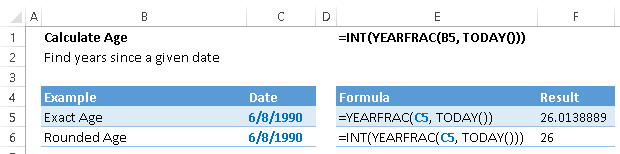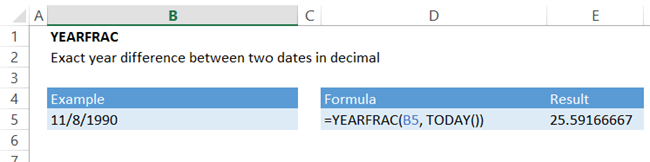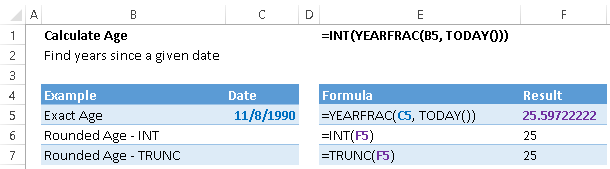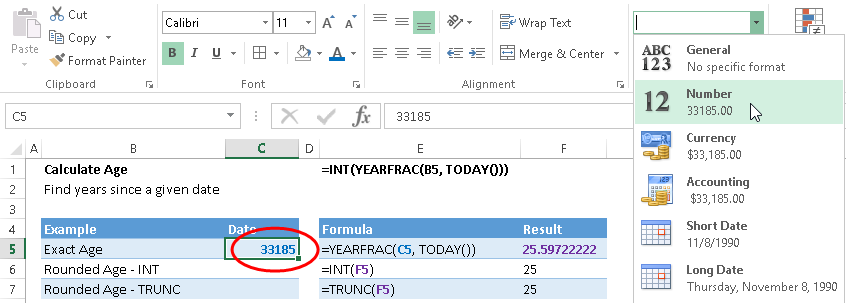# How to Calculate Age in Excel

This tutorial covers how to calculate age in Excel.## Calculate Exact Age

To calculate the exact age of a person, you want to calculate the difference between the current date (or any other desired date) and the birth date.

You might think that you could simply take the difference in days between the current date and the birth date divided by 365: (Current Date – Birth Date) / 365. This answer is close, however, this ignores leap years (Remember that every 4 years, February has an extra day and there are 366 days in that year).

(Current Date – Birth Date)/365.25 will generally produce a more accurate result, however it’s not as precise as it could be. Instead use the YEARFRAC Function:

### YEARFRAC Function

=YEARFRAC(start_date, end_date)

The YEARFRAC Function returns a decimal year difference between two dates.

Look at an example:=YEARFRAC(B5,TODAY())

Note: The TODAY Function: TODAY() returns today’s date (6/13/2016 in our example)

## Calculate Rounded (Truncated) Age

The previous answer was in decimal format (25.59 years). However, we generally would refer to this person as 25 years old, not 25.59 years old.

You can use the INT or TRUNC Functions to simply trim off of decimals (note: you can also use the FLOOR Function, however the Floor Function requires an additional input)=INT(YEARFRAC(C5,TODAY()))

## Dates in Excel

How does Excel do this so easily?

Allow me to show you a secret of how dates work in Excel. Dates in Excel are stored as a value representing the number of days since the first date in Excel.

See for yourself by formatting a date into number formatting in the Home Ribbon.Or by using the shortcut CTRL + SHIFT + 1

November 8, 1990 is 33,185 days since the first day of Excel, the imaginary date of January 0, 1900. So the date 11/8/1990 is stored as the number 33,185. As for dates before the first date, that is a whole another problem for another post!

This tutorial will teach you how to set up and calculate a running total
This tutorial explains how to count the number of cells containing numbers that fall
This tutorial will show you how to compare two numbers and calculate their ratio,
This tutorial will demonstrate various ways to calculate compound interest in Microsoft Excel. What
This tutorial covers how to add entire rows or columns in Excel The Sum